# High School Geometry - Central and Inscribed Angles

## Introduction

• The angle formed by two radii of the circle and having its vertex at the center of the circle is called the central angle.
• In other words, a central angle is an angle subtended by an arc of a circle at the center of the circle, as shown below.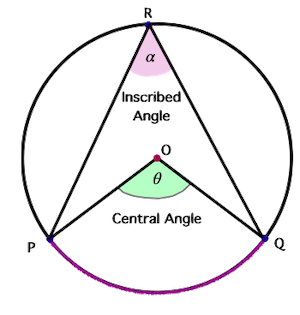• It can be seen that the central angle divides a circle into sectors.
• The angle subtended an arc of a circle at any point on the circumference of the circle is called an inscribed angle.
• In the figure shown above, $\mathbit{\theta }$ is the central angle and $\mathbit{\alpha }$ is the inscribed angle.

## Important Points

• The angle subtended by an arc at the center is double the angle subtended by it at any point on the remaining part of the circle.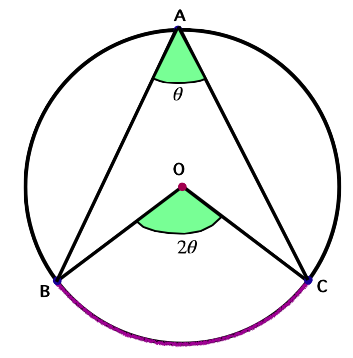• Congruent arcs of a circle subtend equal angles at the center.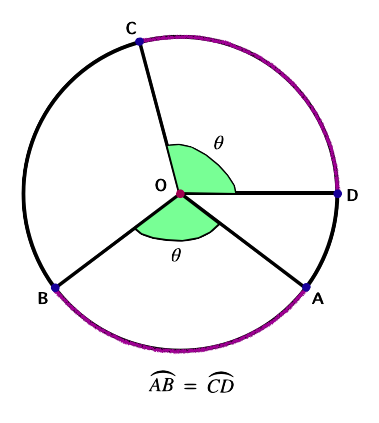• The angle subtended by diameter on any point of a circle is $90°$.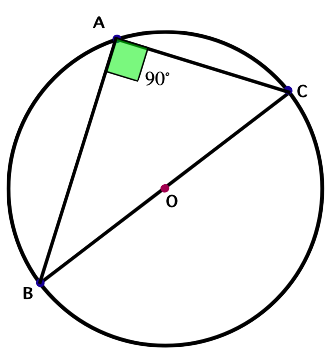• Angles in the same segment of a circle are equal.
• The measure of a central angle is equal to the measure of the arc forming the central angle.
• The measure of an inscribed angle is half the measure of the intercepted arc.
• In a cyclic quadrilateral, the sum of either pair of opposite angles is supplementary.

## Solved Examples

Example 1: Circle O has points A, B, and C on the circle, as shown in the figure. Given $m\angle AOB=50°$, find the measure of $\angle ACB$.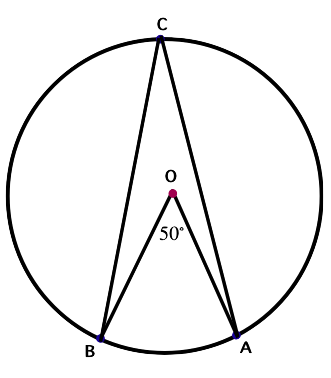Solution: $\angle ACB=\frac{1}{2}\angle AOB$ $=\frac{1}{2}×50°$ $=25°$

Example 2: In the figure shown below $m\stackrel{⏜}{SQ}=45°$ and $\angle PQR=20°$. Determine $m\angle POR$.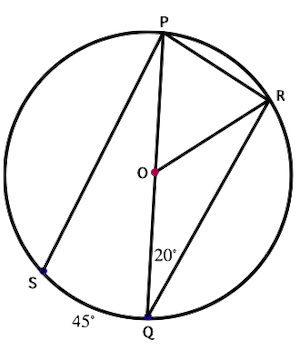Solution:

$\angle OQR=\angle ORQ=20°$

In $∆ROQ$,

$\angle ROQ+\angle OQR+\angle ORQ=180°$

$\angle ROQ+20°+20°=180°$

$\angle ROQ=140°$

Also, $\angle ROQ+\angle POR=180°$

$140°+\angle POR=180°$

$\angle POR=40°$

Question 3: Points A, B, C, and D lie on Circle O. $\angle BAC$ is inscribed in the circle. Find x, given $m\stackrel{⏜}{BDC}=148°$ and $m\angle BAC=\left(4x+24\right)°$.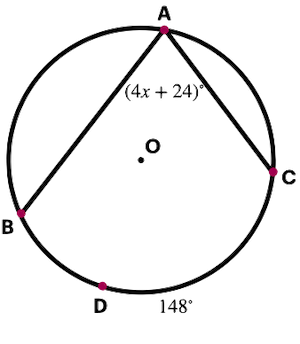Solution: $\stackrel{⏜}{BDC}=148°$$\angle BAC=\left(4x+24\right)°$

$\angle BAC=\frac{1}{2}\stackrel{⏜}{BDC}$

$\left(4x+24\right)°=\frac{1}{2}×148°$

$4x+24°=74°$

$4x=50°$

$x=12.5°$

## Cheat Sheet

• The angle subtended by an arc at the center is double the angle subtended by it at any point on the remaining part of the circle.
• Congruent arcs of a circle subtend equal angles at the center.
• The angle subtended by diameter on any point of a circle is $90°$.
• Angles in the same segment of a circle are equal.
• The measure of an inscribed angle is half the measure of the intercepted arc.
• In a cyclic quadrilateral, the sum of either pair of opposite angles is supplementary.

## Blunder Areas

• The angle subtended by an arc at the center of the circle is equal to the angle measure of the arc.